Hostname: page-component-546b4f848f-fhndm Total loading time: 0 Render date: 2023-05-30T22:21:00.577Z Has data issue: false Feature Flags: { "useRatesEcommerce": true } hasContentIssue false

## Abstract

We define a family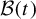$\mathcal {B}(t)$ of compact subsets of the unit interval which provides a filtration of the set of numbers whose continued fraction expansion has bounded digits. We study how the set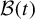$\mathcal {B}(t)$ changes as the parameter t ranges in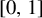$[0,1]$ , and see that the family undergoes period-doubling bifurcations and displays the same transition pattern from periodic to chaotic behaviour as the family of real quadratic polynomials. The set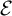$\mathcal {E}$ of bifurcation parameters is a fractal set of measure zero and Hausdorff dimension$1$ . The Hausdorff dimension of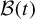$\mathcal {B}(t)$ varies continuously with the parameter, and we show that the dimension of each individual set equals the dimension of the corresponding section of the bifurcation set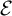$\mathcal {E}$ .

## MSC classification

Type
Original Article
Information
Ergodic Theory and Dynamical Systems , July 2022 , pp. 2239 - 2269

## Access options

Get access to the full version of this content by using one of the access options below. (Log in options will check for institutional or personal access. Content may require purchase if you do not have access.)

## References

Allaart, P.. An algebraic approach to entropy plateaus in non-integer base expansions. Discrete Contin. Dyn. Syst. A 39 (2019), 65076522.CrossRefGoogle Scholar
Barrera, R. Alcaraz, Baker, S. and Kong, D.. Entropy, topological transitivity, and dimensional properties of unique$q$ -expansions. Trans. Amer. Math. Soc. 371 (2019), 32093258.CrossRefGoogle Scholar
Allaart, P. and Kong, D.. On the continuity of the Hausdorff dimension of the univoque set. Adv. Math. 354 (2019), 106729.CrossRefGoogle Scholar
Allaart, P. and Kong, D.. Relative bifurcation sets and the local dimension of univoque bases. Ergod. Th. & Dynam. Sys. doi:10.1017/etds.2020.38. Published online 26 May 2020.CrossRefGoogle Scholar
Bonanno, C., Carminati, C., Isola, S. and Tiozzo, G.. Dynamics of continued fractions and kneading sequences of unimodal maps. Discrete Contin. Dyn. Syst. 33 (2013), 13131332.CrossRefGoogle Scholar
Bruin, H., Carminati, C., Marmi, S. and Profeti, A.. Matching in a family of piecewise affine maps. Nonlinearity 32 (2019), 172208.CrossRefGoogle Scholar
Cassaigne, J.. Limit values of the recurrence quotient of Sturmian sequences. Theoret. Comput. Sci. 218(1) (1999), 312.CrossRefGoogle Scholar
Carminati, C. and Marmi, S.. Linearization of germs: regular dependence on the multiplier. Bull. Soc. Math. France 136(4) (2008), 533564.Google Scholar
Carminati, C. and Tiozzo, G.. A canonical thickening of$\mathbb{Q}$ and the entropy of$\alpha$ -continued fractions. Ergod. Th. & Dynam. Sys. 32(4) (2012), 12491269.CrossRefGoogle Scholar
Carminati, C. and Tiozzo, G.. Tuning and plateaux for the entropy of$\alpha$ -continued fractions. Nonlinearity 26 (2013), 10491070.CrossRefGoogle Scholar
Carminati, C. and Tiozzo, G.. The local Hölder exponent for the dimension of invariant subsets of the circle. Ergod. Th. & Dynam. Sys. 37 (2017), 18251840.CrossRefGoogle Scholar
Das, T., Fishman, L., Simmons, D. and Urbański, M.. Hausdorff dimensions of perturbations of a conformal iterated function system via thermodynamic formalism. Preprint, arXiv:2007.10554.Google Scholar
Douady, A. and Hubbard, J. H.. On the dynamics of polynomial-like mappings. Ann. Sci. Éc. Norm. Supér. (4) 18(2) (1985), 287343.CrossRefGoogle Scholar
Dajani, K. and Kalle, C.. Invariant measures, matching and the frequency of 0 for signed binary expansions. Publ. Res. Inst. Math. Sci. 56(4) (2020), 701742.CrossRefGoogle Scholar
Douady, A.. Systèmes dynamiques holomorphes. Bourbaki Seminar. Vol. 1982/83 (Astérisque, 105). Société Mathématique de France, Paris, 1983, pp. 3963.Google Scholar
Douady, A.. Topological entropy of unimodal maps: monotonicity for quadratic polynomials. Real and Complex Dynamical Systems (Hillerød, 1993) (NATO Advanced Science Institutes Series C: Mathematical and Physical Sciences, 464). Kluwer, Dordrecht, 1995, pp. 6587.CrossRefGoogle Scholar
Erdős, P., Horváth, M. and Joó, I.. On the uniqueness of the expansions$1=\sum {q}^{-{n}_i}$ . Acta Math. Hungar. 58 (1991), 333342.Google Scholar
Falconer, K.. Fractal Geometry: Mathematical Foundations and Applications. Wiley, Chichester, 1990.Google Scholar
Hensley, D.. A polynomial time algorithm for the Hausdorff dimension of continued fraction Cantor sets. J. Number Theory 58(1) (1996), 945.CrossRefGoogle Scholar
Hensley, D.. Continued Fractions. World Scientific, Hackensack, NJ, 2006.CrossRefGoogle Scholar
Hensley, D.. Continued fraction Cantor sets, Hausdorff dimension, and functional analysis. J. Number Theory 40(3) (1992), 336358.CrossRefGoogle Scholar
Jenkinson, O. and Pollicott, M.. Computing the dimension of dynamically defined sets:${E}_2$ and bounded continued fractions. Ergod. Th. & Dynam. Sys. 21 (2001), 14291445.CrossRefGoogle Scholar
Jenkinson, O. and Pollicott, M.. Rigorous effective bounds on the Hausdorff dimension of continued fraction Cantor sets: a hundred decimal digits for the dimension of${E}_2$ . Adv. Math. 325 (2018), 87115.CrossRefGoogle Scholar
Kalle, C., Kong, D., Li, W. and , F.. On the bifurcation set of unique expansions. Acta Arith. 188 (2019), 367399.CrossRefGoogle Scholar
Komornik, V. and Loreti, P.. Unique developments in non-integer bases. Amer. Math. Monthly 105 (1998), 636639.CrossRefGoogle Scholar
Moreira, C. G.. Geometric properties of the Markov and Lagrange spectra. Ann. of Math. 188(1) (2018), 145170.CrossRefGoogle Scholar
Marmi, S. and Sauzin, D.. Quasianalytic monogenic solutions of a cohomological equation. Mem. Amer. Math. Soc. 164(780) (2003), vi+83.Google Scholar
Pollicott, M. and Urbański, M.. Open Conformal Systems and Perturbations of Transfer Operators (Lecture Notes in Mathematics, 2206). Springer, Cham, 2017.CrossRefGoogle Scholar
Raith, P.. Continuity of the Hausdorff dimension for piecewise monotonic maps. Israel J. Math. 80 (1992), 97133.CrossRefGoogle Scholar
Raith, P.. Continuity of the Hausdorff dimension for invariant subsets of interval maps. Acta Math Univ. Comenian. LXIII (1994), 3953.Google Scholar
Tiozzo, G.. Entropy, dimension and combinatorial moduli for one-dimensional dynamical systems. PhD Thesis, Harvard University, 2013.Google Scholar
Tiozzo, G.. Topological entropy of quadratic polynomials and dimension of sections of the Mandelbrot set. Adv. Math. 273 (2015), 651715.CrossRefGoogle Scholar
Tiozzo, G.. The local Hölder exponent for the entropy of real unimodal maps. Sci. China Math. 61(12) (2018), 22992310.CrossRefGoogle Scholar
Urbański, M.. On Hausdorff dimension of invariant sets for expanding maps of a circle. Ergod. Th. & Dynam. Sys. 6(2) (1986), 295309.CrossRefGoogle Scholar